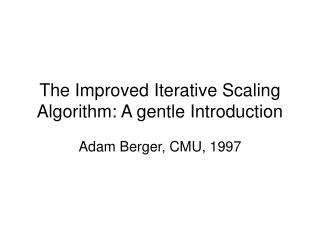DownloadDownload PresentationThe Improved Iterative Scaling Algorithm: A gentle Introduction

# The Improved Iterative Scaling Algorithm: A gentle Introduction

Télécharger la présentation## The Improved Iterative Scaling Algorithm: A gentle Introduction

- - - - - - - - - - - - - - - - - - - - - - - - - - - E N D - - - - - - - - - - - - - - - - - - - - - - - - - - -
##### Presentation Transcript

1. The Improved Iterative Scaling Algorithm: A gentle Introduction Adam Berger, CMU, 1997

2. Introduction • Random process • Produces some output value y, a member of a (necessarily finite) set of possible output values • The value of the random variable y is influenced by some conditioning information (or “context”) x • Language modeling problem • Assign a probability p(y| x) to the event that the next word in a sequence of text will be y, given x, the value of the previous words

3. Features and constraints • The goal is to construct a statistical model of the process which generated the training sample • The building blocks of this model will be a set of statistics of the training sample • The frequency that in translated to either dans or en was 3/10 • The frequency that in translated to either dans or au cours de was ½ • And so on Statistics of the training sample

4. Features and constraints • Conditioning information x • E.g., in the training sample, if April is the word following in, then the translation of in is en with frequency 9/10 • Indicator function • Expected value of f

5. Features and constraints • We can express any statistic of the sample as the expected value of an appropriate binary-valued indicator function f • We call such function a feature function or feature for short

6. Features and constraints • When we discover a statistic that we feel is useful, we can acknowledge its importance by requiring that our modelaccord with it • We do this by constraining the expected value that the model assigns to the corresponding feature function f • The expected value of f with respect to the model p(y | x) is

7. Features and constraints • We constrain this expected value to be the same as the expected value of f in the training sample. That is, we require • We call this requirement a constraint equationor simply a constraint • Finally, we get

8. Features and constraints • To sum up so far, we now have • A means of representing statistical phenomena inherent in a sample of data (namely, ) • A means of requiring that our model of the process exhibit these phenomena (namely, ) • Feature: • Is a binary-value function of (x, y) • Constraint • Is an equation between the expected value of the feature function in the model and its expected value in the training data

9. The maxent principle • Suppose that we are given n feature functions fi, which determine statistics we feel are important in modeling the process. We would like our model to accord with these statistics • That is, we would like p to lie in the subset C of P defined by

10. Exponential form • The maximum entropy principle presents us with a problem in constrained optimization: find the pCwhich maximizes H(p) • Find

11. Exponential form • We maximize H(p) subject to the following constraints: • 1. • 2. • This and the previous condition guarantee that p is a conditional probability distribution • 3. • In other words, p C, and so satisfies the active constraints C

12. Exponential form • To solve this optimization problem, introduce the Lagrangian

13. (2)

14. Maximum likelihood

15. (4)

16. Finding *

17. (5)

18. (6) (7) p(x)q(x)

19. (8)